I.3.1b

4/7/2021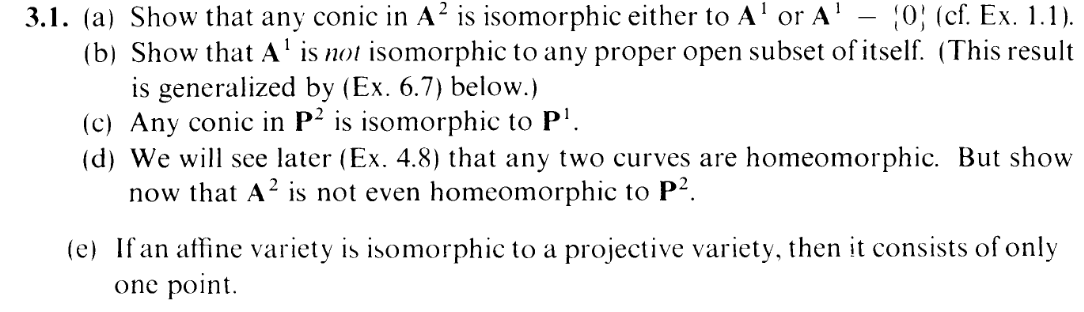Hello. Not an impressive turnout for March, but you know what they say: Never trust a Hartshorner. Our elk graze in bursts, the irregularity governed by whim. Actually, I'm a woodpecker. I peck out my prospective home in bursts, the irregularity governed by whim. Actually, I don't understand how a compound interest works, let alone a mortgage. You'll have to forgive me, reader: I'm currently busy getting buttfucked by conics. Oh, this part of the exercise has nothing to do with conics, sure. But the next part is buttfucking me. It's buttfucking me so hard that I don't even care anymore. I'm starting to self-insert as the girl in Fatalpulse doujinshi, it's great. I'm just living through the stupidity, baby. Yes, we're still on part (b), and the hiatus is slated to continue. I either have to figure it out or look at the solution. Enough time has passed to use the latter, but you know... sunk cost and stubbornness. We've all been there. But hey you know what I think, motherfuckers (bad advice incoming), STAY TRUE TO YOURSELF AND JUST LIVE THROUGH THE STUPIDITY HAHAHAHA.

Anyway, part (c) notwithstanding, I do have the goods for this exercise. Remember Proposition 3.5?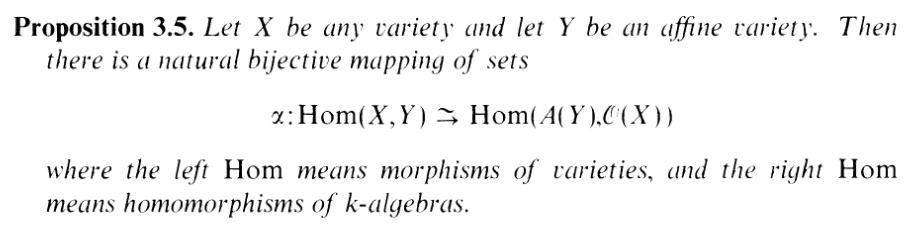Last time, I was uneasy about whether an isomorphism in Hom(A(Y ),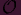(X)) necessarily induced an isomorphism in Hom(X,Y ). After trying to prove it, my guess is that the answer is NO.

However: I figured out that it works in the reverse (in a slightly more general form, even!). Here's a lemma for that:

LEMMA 1:

Given any two varieties X,Y where ϕ : X Y is an isomorphism, then the induced map ψ :(Y )(X) is an isomorphism.

PROOF:

You see, the proof of 3.5 tells us that ψ is given by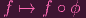(we know that the image is in(X) because ϕ "carries regular functions to regular functions", by virtue of being a morphism) On the other hand, let me define a function

 δ :(X) →(Y ) g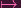g ∘ ϕ-1

Both of these are k-alg homomorphisms and clearly they are inverses, so we're dun.

LEMMA 1 FIN

Now, here's why this is relevant to this exercise. Give me Y = A1 and an open set X Y . Then if there were an isomorphism X Y , then there would have to be an isomorphism A(Y )(X). If I can show the condition of the contrapositive, I'm good. I.e. if I'm able to show A(Y )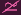(X), I'm good (So I've reduced a "geometric" problem to an "algebraic" problem).

Now obviously A(Y ) = k[x]. What about(X)? Well to figure that out, I'm gonna need another lemma.

I know, there's an assload of bookkeeping here. Here's the thing, folks.(X) are all the regular functions on X, right? Let's review the definition of "regular":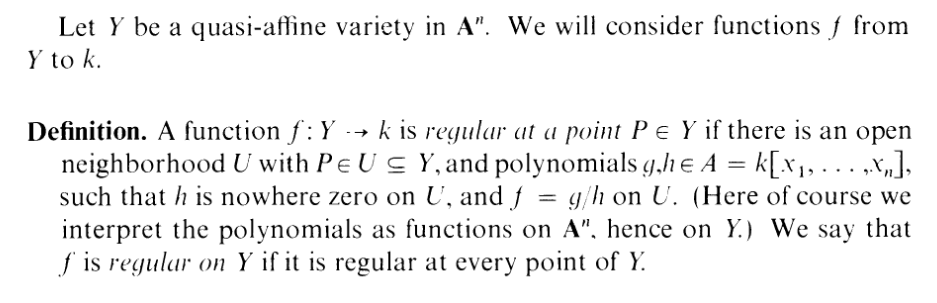Don't you see how complicated these are to classify? A regular function on X isn't just a "rational function". It's a locally rational function. If f(X), I can't just say that f = g∕h. I have to say, oh, f = g∕h in some subset U of X, and maybe f = g∕hin some other subset Uof X, etc. until I cover X with open subsets.

That is so inconvenient. The fact that regular functions are in general not globally rational but locally rational makes the algebra hard. It makes it difficult to calculate what(X) actually is.

However, I found out that in the single dimensional affine case (in our case), regular functions are (globally) rational. Check this out:

LEMMA 2 (Regular funcs on open sets of A1 are rational):

If f(X) where X A1 is open in A1, then as a function on X, f = g∕h on X for g,h k[x] where h nowhere zero. Hence(X) = {rational functions on X}.

PROOF:

We know that f is regular, so it's locally rational. I.e. we may have f = g∕h on U, but f = g∕hon U. How are these two representations of f related? I want to show that they're the same, or at least can be represented as the same rational func.

I know that g∕h = g∕hon U U, so gh′-hg= 0 on U U. I.e. Z(gh′-hg) U U. Now since X is open, by 1.6 I know that X is irreducible. Which means that U Umust be nonempty. And being open sets of not just X, but A1, I know that they're dense in A1. Thus Z(gh′- hg) is a closed sed containing a dense sets of A1, meaning that we must have Z(gh′- hg) = A1. Hence we can write gh= hgon all of A1, which is equivalent to saying that gh= hgin the coordinate ring k[x].

Whew. So that was another "convert the geometry problem into an algebra problem" procedure. Now, readers: How do we compare two polynomials? That's right: Factor em. Since, we're in an algebraically closed field, I can factor g, h, h, gand write

 A(x - g1)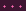(x - ga)B(x - h1′)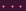(x - hb′) = C(x - g1′)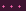(x - gc′)D(x - h1)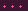(x - hd)

And since this factorization is unique, I know that AB = CD, and the linear factors on the left must match up with the linear factors on the right, perhaps permuted.

Now, in the case that a = c and (after a possible repermutation) the gis match up with the gis, I can actually conclude that g = gand therefore h = h, and thus g∕h = g∕h, which is what I want. But what if the gis and gis don't match up? What if, say, there's an (x - g1) on the left hand side but there's no i such that g1 = gi. Since the factorization is unique, I then know that then g1 has to match up with one of the his. Repermuting it so that it's i = 1, I can say that (x - g1) = (x - h1)... But this just means that (x - g1) appears in the numerator of g∕h and (x - h1) appears in the denominator, so I can cancel them out to get a new rational expression g∕h, where certainly h0 on U (since I'm essentially getting rid of a hole) and f = g∕h on U. So what do I do? Just keep cancelling out elements until g∕h = g∕h.

Hence, if f has a rational representaion on U and a separate rational representation on U, I can assume without loss of generality that it has a rational representation on U U: f = g∕h on U U. Alright, that's good. But I want f to be globally rational. I want to write f = g∕h on all of X.

If I start with U, I can glob up another Uso f is rational on U U. And then maybe from there, I can glob up another U′′, and then after that another U′′′, so f is rational on U U′∪ U′′U′′′. But that still might not constitute all of X, like I want. Uh oh.

Well, by the definition of regularity, I know that there's a UP at least for each point P in X, so these UPs form an open cover of X, but again, that maybe infinitely many open sets to glob up, so we're still stuck at the same problem.

Can I, perhaps, make a finite subcover then? If X were just quasi-compact, then I would be done, (since I can certainly glob up a finite amount of open sets). So, is X quasi-compact? Well, from 1.7c, I know that since A1 is Noetherian, X is at least Noetherian. But then 1.7b tells me that X then has to be quasi-compact.

SO LEMMA 2 DUN.

Actually, while writing this, I realized that pretty much the exact same argument works for any open set X An (since k[x1,,xn] is a UFD). n doesn't have to be 1. So if X is an open set of An, regular functions are rational functions. NOTE: This is not the same thing as saying "if X is quasi affine, regular functions are rational functions". Quasi-affine varieties are sets open with respect to any variety. So if, e.g., all we knew about X were that it was an open set of some variety Z(f), we wouldn't be able to make the same argument (the part in the proof where I claim the zero set of the polynomials are dense in An is where the proof would fail). BTW: I honestly forgot for a while that quasi-affine meant not just open in An but open in any variety, which caused some major confusions while thinking about this lemma.

OKAY. Back to the main thread of the proof. Errr... what were we doing? Right. I wanted to show that A(Y )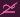(X) and I was trying to compute what(X) was. Now I know that it's simply all the rational functions whose denominators are nonzero on X. I.e.(X) = S-1k[x], the localization of a ring where S = {h|h0onX}.

So our problem reduces to showing that, in general, k[x]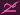S-1k[x], for Sk -{0} (because this is just the case where S-1k[x] = k[x] i.e. X = A1)

Alright. Let's say we had a k-algebra isomorphism

 ψ : k[x] → S-1k[w] x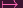g∕h

Let δ = ψ-1. So we must have

 δ(g∕h) = xδ(g)δ(1∕h) = x

So between δ(g) and δ(1∕h), one of them has to have degree 0 (but neither have a value of 0) and the other has to have degree 1.

CASE: deg δ(g) = 0

Let's say deg δ(g) = C where C k (C0). Then

 Cδ(1∕h) = x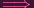δ(1∕h) = (1∕C)xδ(1∕h)δ(h) = (1∕C)xδ(h)δ(1) = (1∕C)xδ(h)1 = (1∕C)xδ(h)

which is a contradiction because x is not invertible in k[x]. CASE 1 DUN.

CASE 2: deg δ(1∕h) = 0

So this time δ(1∕h) = C for some C0 in k, and

 δ(g)C = x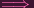δ(g) = (1∕C)x

Now, from this point, note that I can't just multiply by "δ(1∕g)" on both sides because 1∕g might not actually be an element in(X)! (g might not be in S).

on the other hand, remember that δ is a k-alg isomorphism. And since polynomials over (1∕C)x generate all of k[x], polynomials over ψ((1∕C)x) = g generate all of S-1k[x]. In particular, for a given degree 1 polynomial in S, say, f (at least one such polynomial exists, since we assumed Sk -{0}, we would have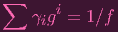which, clearly, is impossible. CASE 2 DUN.

And that's the end of this proof. Note that one consequnce of this is that affine parabolas are NOT isomorphic to hyperbolas/ellipses (since parabolas are A1 and hyperbolas are A1 - 0)

See you NOT soon, as I cannot seem to unbuttfuck myself out of part (c). But I will be back. Keep an eye out, like those black sheaf symbols.